# Introduction

To group tracks with similar properties, one can in principle perform any clustering method of interest on a feature matrix of quantification metrics for each track in the dataset. The package comes with three convenience functions – getFeatureMatrix(),trackFeatureMap(), and clusterTracks() – to easily compute several metrics on all tracks at once, visualize them in 2 dimensions, and to cluster tracks accordingly. This tutorial shows how to use these functions to explore heterogeneity in a track dataset.

# Datasets

library( celltrackR )


The package contains a dataset of T cells imaged in a mouse peripheral lymph node using two photon microscopy. We will here use this dataset as an example of how to perform quality control.

The dataset consists of 21 tracks of individual cells in a tracks object:

str( TCells, list.len = 2 )
#> List of 22
#>  $0 : num [1:11, 1:4] 0 27.8 55.5 83.3 111.1 ... #> ..- attr(*, "dimnames")=List of 2 #> .. ..$ : chr [1:11] "1" "2" "3" "4" ...
#>   .. ..$: chr [1:4] "t" "x" "y" "z" #>$ 1 : num [1:11, 1:4] 0 27.8 55.5 83.3 111.1 ...
#>   ..- attr(*, "dimnames")=List of 2
#>   .. ..$: chr [1:11] "1" "2" "3" "4" ... #> .. ..$ : chr [1:4] "t" "x" "y" "z"
#>   [list output truncated]
#>  - attr(*, "class")= chr "tracks"


Each element in this list is a track from a single cell, consisting of a matrix with $$(x,y,z)$$ coordinates and the corresponding measurement timepoints:

head( TCells[] )
#>         t       x       y    z
#> 1   0.000 132.521 118.692 8.75
#> 2  27.781 133.909 118.700 8.75
#> 3  55.484 131.763 118.129 6.25
#> 4  83.296 133.161 117.903 6.25
#> 5 111.093 131.530 117.894 6.25
#> 6 138.906 132.229 117.665 6.25


Similarly, we will also use the BCells and Neutrophils data:

str( BCells, list.len = 2 )
#> List of 24
#>  $0 : num [1:18, 1:4] 27.8 55.5 83.3 111.1 138.9 ... #> ..- attr(*, "dimnames")=List of 2 #> .. ..$ : chr [1:18] "1" "2" "3" "4" ...
#>   .. ..$: chr [1:4] "t" "x" "y" "z" #>$ 1 : num [1:30, 1:4] 0 27.8 55.5 83.3 111.1 ...
#>   ..- attr(*, "dimnames")=List of 2
#>   .. ..$: chr [1:30] "19" "20" "21" "22" ... #> .. ..$ : chr [1:4] "t" "x" "y" "z"
#>   [list output truncated]
#>  - attr(*, "class")= chr "tracks"
str( Neutrophils, list.len = 2 )
#> List of 10
#>  $0: num [1:25, 1:4] 28.2 56.4 84.6 113.1 141.3 ... #> ..- attr(*, "dimnames")=List of 2 #> .. ..$ : chr [1:25] "1" "2" "3" "4" ...
#>   .. ..$: chr [1:4] "t" "x" "y" "z" #>$ 1: num [1:22, 1:4] 0 28.2 56.4 84.6 113.1 ...
#>   ..- attr(*, "dimnames")=List of 2
#>   .. ..$: chr [1:22] "26" "27" "28" "29" ... #> .. ..$ : chr [1:4] "t" "x" "y" "z"
#>   [list output truncated]
#>  - attr(*, "class")= chr "tracks"


Combine them in a single dataset, where labels also indicate celltype:

T2 <- TCells
names(T2) <- paste0( "T", names(T2) )
tlab <- rep( "T", length(T2) )

B2 <- BCells
names(B2) <- paste0( "B", names(B2) )
blab <- rep( "B", length(B2) )

N2 <- Neutrophils
names(N2) <- paste0( "N", names(Neutrophils) )
nlab <- rep( "N", length( N2) )

all.tracks <- c( T2, B2, N2 )
real.celltype <- c( tlab, blab, nlab )


# 1 Extracting a feature matrix

Using the function getFeatureMatrix(), we can quickly apply several quantification measures to all data at once (see ?TrackMeasures for an overview of measures we can compute):

m <- getFeatureMatrix( all.tracks,
c(speed, meanTurningAngle,
outreachRatio, squareDisplacement) )

# We get a matrix with a row per track and one column for each metric:
#>          [,1]      [,2]      [,3]        [,4]
#> T0 0.04111888 2.5748446 0.2458866    7.389989
#> T1 0.16407805 0.6500093 0.8004166 1330.130666
#> T2 0.14649521 1.0933954 0.4130841 1590.395966
#> T3 0.12828778 1.2933856 0.4102647 2994.020167
#> T4 0.08589151 1.4616603 0.5382724  695.435841
#> T5 0.26304168 0.7005672 0.4257547 1636.184659


We can use this matrix to explore relationships between different metrics. For example, we can observe a negative correlation between speed and mean turning angle:

plot( m, xlab = "speed", ylab = "mean turning angle" )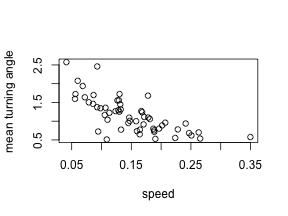# 2 Dimensionality reduction methods: PCA, MDS, and UMAP

When using more than two metrics at once to quantify track properties, it becomes hard to visualize which tracks are similar to each other. Like with single-cell data, dimensionality reduction methods can help visualize high-dimensional track feature datasets. The function trackFeatureMap() is a wrapper method that helps to quickly visualize data using three popular methods: principal component analysis (PCA), multidimensional scaling (MDS), and uniform manifold approximate and projection (UMAP). The function trackFeatureMap() can be used for a quick visualization of data, or return the coordinates in the new axis system if the argument return.mapping=TRUE.

### 1.1 PCA

Use trackFeatureMap() to perform a principal component analysis (PCA) based on the measures “speed” and “meanTurningAngle”, using the optional labels argument to color points by their real celltype and return.mapping=TRUE to also return the data rather than just the plot:

pca <- trackFeatureMap( all.tracks,
c(speed,meanTurningAngle,squareDisplacement,
maxDisplacement,outreachRatio ), method = "PCA",
labels = real.celltype, return.mapping = TRUE )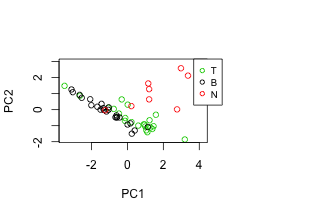We can then inspect the data stored in pca. This reveals, for example, that the first principal component is correlated with speed:

pc1 <- pca[,1]
pc2 <- pca[,2]
track.speed <- sapply( all.tracks, speed )
cor.test( pc1, track.speed )
#>
#>  Pearson's product-moment correlation
#>
#> data:  pc1 and track.speed
#> t = 9.2314, df = 54, p-value = 1.072e-12
#> alternative hypothesis: true correlation is not equal to 0
#> 95 percent confidence interval:
#>  0.6540020 0.8669588
#> sample estimates:
#>       cor
#> 0.7823818


See ?prcomp for details on how principal components are computed, and for further arguments that can be passed on to trackFeatureMap().

### 1.2 MDS

Another popular method for visualization is multidimensional scaling (MDS), which is also supported by trackFeatureMap():

trackFeatureMap( all.tracks,
c(speed,meanTurningAngle,squareDisplacement,maxDisplacement,
outreachRatio ), method = "MDS",
labels = real.celltype )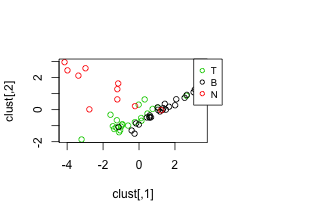Internally, trackFeatureMap() computes a distance matrix using dist() and then applies MDS using cmdscale(). See the documentation of cmdscale for details and further arguments that can be passed on via trackFeatureMap().

### 1.3 UMAP

Uniform manifold approximate and projection (UMAP) is another powerful method to explore structure in high-dimensional datasets. trackFeatureMap() supports visualization of tracks in a UMAP. Note that this option requires the uwot package. Please install this package first using install.packages("uwot").

trackFeatureMap( all.tracks,
c(speed,meanTurningAngle,squareDisplacement,
maxDisplacement,outreachRatio ), method = "UMAP",
labels = real.celltype )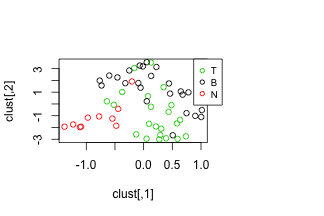# 3 Clustering: hierarchical clustering and k-means

To go beyond visualizing similar and dissimilar tracks using multiple track features, clusterTracks() supports the explicit grouping of tracks into clusters using two common methods: hierarchical and k-means clustering.

### 3.1 Hierarchical clustering

Hierarchical clustering is performed by calling hclust() on a distance matrix computed via dist() on the feature matrix:

clusterTracks( all.tracks,
c(speed,meanTurningAngle,squareDisplacement,maxDisplacement,
outreachRatio ), method = "hclust", labels = real.celltype )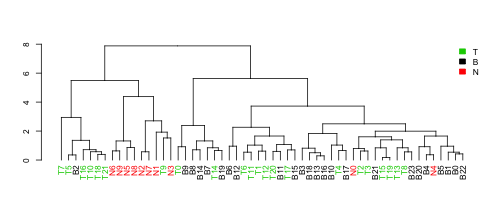See methods dist() and hclust() for details.

### 3.2 K-means clustering

Secondly, clusterTracks() also supports k-means clustering of tracks. Note that this requires an extra argument centers that is passed on to the kmeans() function and specifies the number of clusters to make. In this case, let's use three clusters because we have three celltypes:

clusterTracks( all.tracks,
c(speed,meanTurningAngle,squareDisplacement,maxDisplacement,
outreachRatio ), method = "kmeans",
labels = real.celltype, centers = 3 )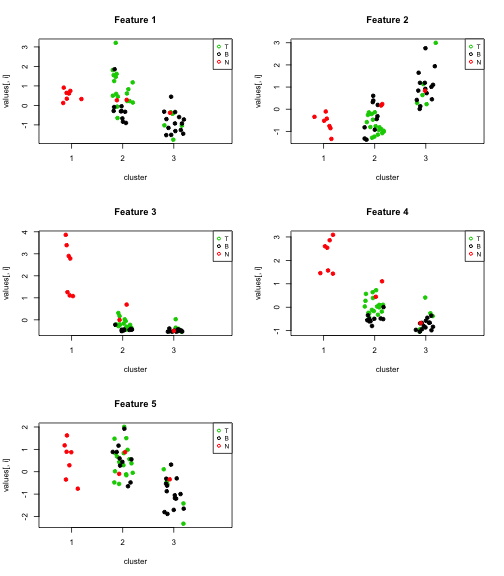In these plots, we see the value of each feature in the feature matrix plotted for the different clusters, whereas the color indicates the “real” celltype the track came from.